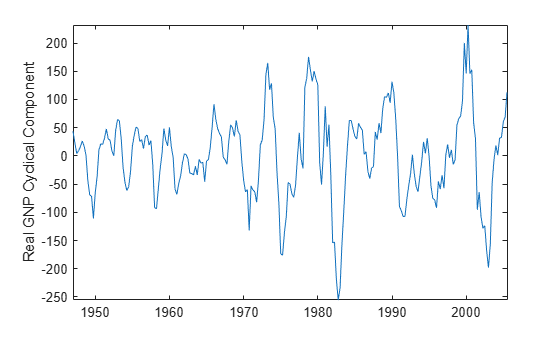# hpfilter

Hodrick-Prescott filter for trend and cyclical components

## Syntax

``hpfilter(Y)``
``hpfilter(Y,smoothing)``
``Trend = hpfilter(___)``
``[Trend,Cyclical] = hpfilter(___)``

## Description

example

``hpfilter(Y)` plots the data of the time series variables (columns) of `Y` and their respective trend components computed by the Hodrick-Prescott Filter. The smoothing parameter is `1600`, which is appropriate for quarterly periodicity. `hpfilter` plots all time series and their respective trend components on the same axes.`
``hpfilter(Y,smoothing)` applies the Hodrick-Prescott filter smoothing parameter `smoothing`.`
``Trend = hpfilter(___)` returns the trend components `Trend` of the time series variables using any of the input argument combinations in the previous syntaxes.`
``[Trend,Cyclical] = hpfilter(___)` also returns the cyclical components `Cyclical`.`

## Examples

collapse all

Plot the cyclical component of the US post-WWII seasonally-adjusted real gross national poroduct (GNP). Specify `smoothing` of 1600, which is appropriate for quarterly data.

```load Data_GNP gnpDate = dates; realgnp = DataTable.GNPR; [~,c] = hpfilter(realgnp,1600); plot(gnpDate,c) axis tight ylabel('Real GNP cyclical component')```## Input Arguments

collapse all

Time series data, specified as a numeric vector of length `numObs` or a `numObs`-by-`numSeries` numeric matrix.

• A vector represents `numObs` observations of a single series or variable.

• A matrix represents `numObs` observations of `numSeries` series. `Y(j,k)` is the observed value of series `k` at time `j`. Observations within the same row occur simultaneously.

The last element or row contains the latest observation.

If any element of `Y` is `NaN` or `Inf`, `hpfilter` issues an error.

Data Types: `double`

Trend component smoothing parameter, specified as a nonnegative numeric scalar or a nonnegative numeric vector of length `numSeries`. For a numeric scalar, `hpfilter` applies `smoothing` to all series in `Y`. For a numeric vector, `hpfilter` applies `smoothing(k)` to series `k` in the data (`Y(:,k)`).

If `smoothing(k)` is `0`, `hpfilter` does not smooth the trend component of series `k`. In this case, the following are true:

• `Trend(:,k)` = `Y(:,k)`.

• `Cyclical(:,k)` = `zeros(numObs,1)`.

If `smoothing(k)` is `Inf`, `hpfilter` applies the maximum smoothing. In this case, the following are true:

• `Trend(:,k)` is the linear time trend computed by least squares.

• `Cyclical(:,k)` is the detrended series.

As the magnitude of the smoothing parameter increases, `Trend` approaches the linear time trend.

Appropriate values of the smoothing parameter depend on the periodicity of the data. Although a best practice is to experiment with smoothing values for your data, these smoothing values are recommended :

• `14400` for monthly data

• `1600` for quarterly data

• `100` for yearly data

Example: `100`

Data Types: `double`

## Output Arguments

collapse all

Trend component τt of each series in the data, returned as a numeric vector or matrix with the same dimensions as `Y`.

Cyclical component ct of each series in the data, returned as a numeric vector or matrix with the same dimensions as `Y`.

collapse all

### Hodrick-Prescott Filter

The Hodrick-Prescott filter decomposes an observed time series yt (`Y`) into a trend component τt (`Trend`) and a cyclical component ct (`Cyclical`) such that yt = τt + ct.

The objective function of the filter is

`$f\left({\tau }_{t}\right)=\sum _{t=1}^{T}{\left({y}_{t}-{\tau }_{t}\right)}^{2}+\lambda \sum _{t=2}^{T-1}{\left[\left({\tau }_{t+1}-{\tau }_{t}\right)-\left({\tau }_{t}-{\tau }_{t-1}\right)\right]}^{2},$`

where:

The programming problem is to minimize the objective function over τ1,…,τT. The objective penalizes the sum of squares for the cyclical component with the sum of squares of second-order differences for the trend component (trend acceleration penalty). If λ = 0, the minimum of the objective is 0 with τt = yt for all t. As λ increases, the penalty for a flexible trend increases, resulting in an increasingly smoother trend. When λ is arbitrarily large, the trend acceleration approaches 0, resulting in a linear trend.

This figure shows the effects of increasing the smoothing parameter on the trend component for a simulated series.The filter is equivalent to a cubic spline smoother, where the smoothed component is τt.

## Tips

• For high-frequency series, the Hodrick-Prescott filter can produce anomalous endpoint effects. In this case, do not extrapolate the series using the results of the filter.

 Hodrick, Robert J., and Edward C. Prescott. "Postwar U.S. Business Cycles: An Empirical Investigation." Journal of Money, Credit and Banking 29, no. 1 (February 1997): 1–16. https://doi.org/10.2307/2953682.